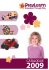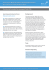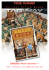# How to make a picturesque maze 1 Introduction

## Transcription

How to make a picturesque maze 1 Introduction
```CCCG 2009, Vancouver, BC, August 17–19, 2009
How to make a picturesque maze
Yoshio Okamoto∗†
Abstract
In the picturesque maze generation problem, we are
given a rectangular black-and-white raster image and
want to randomly generate a maze in which the solution path fills up the black pixels. While a simple formulation of the problem faces with NP-hardness, the
proposed method generates such a maze in polynomial
time by appropriately changing the formulation itself.
Therefore, the algorithm itself is quite simple.
Ryuhei Uehara‡
1
Introduction
The goal of this work is to solve the following picturesque maze generation problem.
Picturesque Maze Generation Problem
Input A black-and-white raster image with m rows and
n columns.
Output A maze in which the solution path fills up the
input black pixels.
Figure 1 shows an example. The specification of an
input and an output is vague: This is intentional. Refinement of this part is contained in the formulation (or
the formalization) of the problem, and this also forms a
core of this work.
Our formalization and the algorithm have the following features.
• We only require the input image to be connected
with respect to the 4-neighborhood1 (good).
• The solution path of an output precisely coincides
with the input image (good).
• We do not face with any NP-hard subproblems, and
the core subroutine is random generation of a spanning tree in an undirected graph. Therefore, we do
not need any complicated algorithm (good).
• We cannot specify the entrance and the exit arbitrarily (bad).
• The quality of an output maze does not have to be
Figure 1: An example of a picturesque maze. (Up) A
given black-and-white raster image. (Middle) An output, where two green rhombi represent the entrance and
the exit. (Down) Showing that the solution path gives
the input image.
∗ Graduate School of Information Science and Engineering,
Tokyo Institute of Technology, [email protected]
† Supported by Global COE Program “Computationism as a
Foundation for the Sciences” and Grant-in-Aid for Scientific Research from Ministry of Education, Science and Culture, Japan,
and Japan Society for the Promotion of Science.
‡ School of Information Science, Japan Advanced Institute of
Science and Technology, [email protected]
Maze generation is a subfield of puzzle generation,
and it strongly has an artistic aspect. There are some
algorithmic studies on maze generation. First, it often appears as an application of random generation of
spanning trees, and for example a paper by Propp and
Wilson  exhibits a randomly generated maze. The
webpage “Think Labyrinth!” by Walter D. Pullen 
explains the outlines of many maze generation algorithms. However, these algorithms do not generate a
picturesque maze.
Not much research has been done for generation of
picturesque mazes. For mazes that show pictures as
their solutions, as in this work, Conceptis Ltd. developed a generation algorithm, and published several
books with lots of picturesque mazes made by it (that
1 Equivalent to the von Neumann neighborhood in the field of
cellular automata.
21st Canadian Conference on Computational Geometry, 2009
they call “Maze-a-Pix”, for example see ). The detail
of their algorithm is not made public to us. There are
also some Japanese books on picturesque mazes [6, 11],
but they do not look to be automatically generated. On
the other hand, Xu and Kaplan [9, 10] study the automatic generation of mazes that themselves look like
pictures.
2
Outline of our method
For a given black-and-white raster image with m rows
and n columns, we call the set of black pixels the foreground, and the set of white pixels the background.
First, let us review how to generate a (not necessarily picturesque) maze. We are given a rectangle with m
rows and n columns (Figure 2 up left). We think of each
cell as a vertex (Figure 2 up middle), and join a pair of
adjacent cells by an edge (Figure 2 up right). This gives
rise to an undirected graph. Then, choose a spanning
tree of the graph (Figure 2 down left). Remove the edges
of cells that cross the edges of the spanning tree (Figure 2 down middle), and choose arbitrary two cells as
the entrance and the exit. This completes the construction of a maze (Figure 2 down right). A path between
the entrance and the exit is uniquely determined, and
this is the solution path of the maze.
Figure 2: How to construct a maze.
Now, let us move to generation of a picturesque maze.
We are given a black-and-white raster image with m
rows and n columns (Figure 3 left). As before, we construct an undirected graph by thinking of each cell as
a vertex (Figure 3 middle), and a pair of adjacent cells
as an edge (Figure 3 right). If we could choose an appropriate spanning tree of this graph, we would obtain
a desired maze. However, to this end, the graph should
possess a path that goes through all vertices corresponding to the black cells. Namely, if we call the subgraph
induced by the vertices corresponding to the black cells
the foreground subgraph, then such a path is a Hamiltonian path of the foreground subgraph.
Unfortunately, an input image might have no such
Hamiltonian path. Furthermore, it is NP-complete to
decide whether such a graph (namely, a grid graph) has
a Hamiltonian path . If the input image has no “hole”
Figure 3: Toward generation of a picturesque maze.
(namely, the image is simply connected), then this decision problem can be solved in polynomial time .
However, it would be a quite strong assumption if we
would forbid the existence of a hole, and even though the
image has no hole, we cannot guarantee the existence
of a Hamiltonian path. If the foreground subgraph has
no Hamiltonian path, then we need to modify the input
image. We would need to do all of these tasks efficiently
while keeping the quality of the image, and this is not
trivial.
Hence, in this work we only require the input image to
give rise to a connected foreground subgraph. If it is not
connected, we still need to modify the input; However,
this is much easier than making the graph have a Hamiltonian path. The connectedness of an undirected graph
can be decided in linear time (see an appropriate textbook on graph algorithms). There is even a linear-time
constant-space algorithm to decide the connectedness of
a black-and-white raster image .
Our method can be summarized in the following sentence: If the foreground subgraph has no Hamiltonian
path, then, instead of modifying the image to have a
Hamiltonian path, we just modify the scale of the image.
Here is a more concrete description. Again, we are given
a black-and-white image with m rows and n columns
(Figure 4 left). Then, we subdivide each cell into 2 × 2
smaller cells. This yields an image with 2m rows and 2n
columns (Figure 4 middle). If the foreground subgraph
of the original input image is connected, then the foreground subgraph of the new finer image always has a
Hamiltonian path, and such a path can be found quite
easily as explained in the next section. Based on this
path, we can generate a desired maze (Figure 4 right).
This is the outline. The next section explains the detail.
Figure 4: Outline of our method.
3
Detail of our method
Our method is divided into the following two steps.
CCCG 2009, Vancouver, BC, August 17–19, 2009
3.1
Generation of the foreground or a solution path
Again, we are given a black-and-white image with m
rows and n columns in which the foreground graph is
connected (Figure 5 up left). We construct the undirected graph as before (Figure 5 up middle). In the
foreground graph (Figure 5 up right), we generate a
random spanning tree (Figure 5 down left). Now we
traverse the spanning tree in the planar manner (Figure 5 down middle). Then, along the traversal path we
create a Hamiltonian path in the refined image with 2m
rows and 2n columns. This forms a solution path of our
maze (Figure 5 down right). The entrance and the exit
of the maze will be the endpoints of the Hamiltonian
path.
Figure 5: Generation of the foreground.
3.2
Generation of the background
Now we look at the refined image with 2m rows and 2n
columns and consider the subgraph obtained by removing the edges in the foreground graph that are not used
in the Hamiltonian path (Figure 6 left). Generate a random spanning tree in that subgraph (Figure 6 middle),
and extract the maze from the spanning tree (Figure 6
right).
This completes the description of our generation algorithm for picturesque mazes. It is simple. The complexity depends on how to generate a random spanning
tree, but it can be done in polynomial time (e.g. ).
without solving the mazes. To avoid this, we introduce
a simple heuristic improvement method.
As a reason for the separation, we observed that the
foreground is often coarser than the background. This
seems because the foreground is produced from the m ×
n grid while the background is from the 2m × 2n grid.
Therefore, it is important to erase a fine structure of
a background. One of such fine structures is a group
darker when seen from the distance. Furthermore, a
maze with many deadends is easier to solve since it is
easy to detect a wrong branch at an intersection of the
maze: Such a maze is not fun. Hence, it should be
effective to introduce a heuristic method to reduce the
another deadend. A concrete procedure is as follows.
In the maze, let two cells u and v be adjacent deadends that are not the entrance or the exit. Then, let
Pu be a path from u to the first intersection bu in the
maze. Similarly, let Pv be a path from v to the first
intersection bv (Figure 7 left). Now, for example, if at
the intersection bu we put a wall in front of the path
Pu , and destroy the wall between u and v, then we obtain another maze (Figure 7 right). In this new maze,
v is not a deadend any more since we have a path from
the new wall at the end of Pu to bv through Pu , u, v
and Pv . This operation always decreases the number of
v
3.3
Heuristic improvement
From the preliminary implementation, we observed that
in some large mazes generated by our method the foreground and the background can easily be separated
v
u
u
bv
bv
Figure 7: A heuristic improvement of a maze.
As a heuristic method, we repeat this operation until
no more operation can be applied. By a clever implementation, this can be done in linear time (i.e., O(mn)
time). In fact Figure 1 is the outcome of this improvement. Figure 8 shows a successive application of this
heuristic operations to the maze in Figure 6 right.
4
Figure 6: Generation of the background.
bu
bu
A little bigger example
For fun, we made a little bigger sample maze in Figure 9.
In the first row you can find the entrance and the exit.
5
Conclusion
We proposed a method to generate a random picturesque maze for a given black-and-white raster image.
21st Canadian Conference on Computational Geometry, 2009
 Conceptis Limited. Conceptis puzzles.
http://www.conceptispuzzles.com/, Accessed on July
1, 2009.
 Conceptis Puzzles. Picture This! Mazes. Sterling, New
York, 2005.
 A. Itai, C.H. Papadimitriou and J.L. Szwarcfiter.
Hamilton paths in grid graphs. SIAM Journal on Computing 11 (1982) 676–686.
 W. Lenhart and C. Umans. Hamiltonian cycles in solid
grid graphs. Proc. 38th FOCS (1997) 496–507.
 S. Mochizuki. Ukidashi Meiro 1 (Japanese). Gakken,
2006.
 J.G. Propp, D.B. Wilson. How to get a perfectly random sample from a generic Markov chain and generate
a random spanning tree of a directed graph. Journal of
Algorithms 27 (1998) 170–217.
 W.D. Pullen. Think Labyrinth!
http://www.astrolog.org/labyrnth.htm, Accessed on
July 1, 2009.
 J. Xu and C.S. Kaplan. Image-guided maze construction. Proceedings of SIGGRAPH 2007, ACM Transactions on Graphics 26 (2007), Article No. 29.
 J. Xu and C.S. Kaplan. Vortex maze construction. Journal of Mathematics and the Arts 1 (2007) 7–20.
 K. Yuzawa. Aiueo Meiro (Japanese). Nikoli, 2003.
Figure 8: Successive application of our heuristic operations. A pair of blue crosses means the deadends to
which we apply the operation.
Our method is simple and easy to implement. In practice it is fast, so we plan to exhibit a demonstration at
the conference.
The world of maze construction looks deep, and more
algorithmic problems should be hidden. It has a strong
artistic flavor, and so we need to utilize methods both
from computer graphics and from algorithm theory.
Acknowledgment The authors thank Ryohei Nakai
for implementing the preliminary algorithm which
shows interesting phenomena.
References
 T. Asano and H. Tanaka. Constant-working space algorithms for connected components labeling (Japanese).
COMP2008-1 (2008) pp. 1–8.
Figure 9: A sample maze with 196 rows and columns.
Namely, the input image has 98 rows and columns.
```

### Corn Maze - the Greater Maple Valley Community Center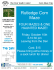### 8 fun things to do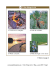### Edmonton Corn Maze 780-288-0208 / 780-554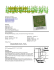### Newsletter 28: 22 May 2013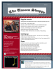### Emergence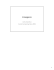### Fall 2015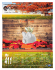### File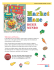### construction - play